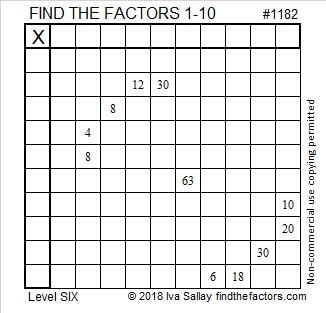# 1182 A Unique Solution

Every Find the Factors puzzle I make has a unique solution. That fact is central to the logic needed to begin this particular puzzle. I hope it frustrates you a little, but not too much. Then when you finally solve it, it will be so much sweeter!Print the puzzles or type the solution in this excel file: 10-factors-1174-1186

Here are some facts about the number 1182:

• 1182 is a composite number.
• Prime factorization: 1182 = 2 × 3 × 197
• The exponents in the prime factorization are 1, 1, and 1. Adding one to each and multiplying we get (1 + 1)(1 + 1)(1 + 1) = 2 × 2 × 2 = 8. Therefore 1182 has exactly 8 factors.
• Factors of 1182: 1, 2, 3, 6, 197, 394, 591, 1182
• Factor pairs: 1182 = 1 × 1182, 2 × 591, 3 × 394, or 6 × 197
• 1182 has no square factors that allow its square root to be simplified. √1182 ≈ 34.380231182 is the hypotenuse of a Pythagorean triple:
168-1170-1182 which is 6 times (28-195-197)

1182 is a palindrome in two bases:
It’s 606 in BASE 14 because 6(14²) + 6(1) = 6(197) = 1182,
and 2J2 in BASE 20 (J is 19 base 10) because 2(20²) + 19(20) + 2(1) = 1182

This site uses Akismet to reduce spam. Learn how your comment data is processed.